Algebraic Approximations of Ellipse Perimeters and of the Complete Elliptic Integral E(x) by Stanislav Sýkora, Extra Byte, Via R.Sanzio 22C, Castano Primo, Italy 20022 in Stan's Library, Ed.S.Sykora, Vol.I. First release June 6, 2006 Permalink via DOI:  10.3247/SL1Math06.004
 Other math articles in Stan's Library | SCIENCE Links Stan's Courses | Stan's HUB
 Abstract This Note builds on the previous work by the Author as well as the recent advances made by W.Cantrell. It starts from stating the obvious, namely that any purely algebraic approximation (i.e., one which uses only the four basic operations of the real numbers algebra) to the ellipse perimeter can be written as a rational function in terms of the ellipse semiaxes a and b. Applying the requirements of (i) symmetry under the permutation of the pair (a,b), (ii) correct scaling behavior, (iii) exact result in known extreme cases and (iv) smallest possible maximum relative error, the number of adjustable coefficients in each admissible expression is drastically reduced, leaving a progression of approximations, each of which is completely defined by the degree of the rational function denominator (the approximation order n). Some of the approximations, particularly those corresponding to n = 1, 3 and 5, coincide with known formulae, while the approximations obtained for n = 2, 4, 6 and 7 appear to be new. Of these, the last two are particularly interesting since their maximum relative errors are better than 0.988 ppm and 0.282 ppm, respectively, which are new records. Rational approximations corresponding to n > 7 would be doubtless even better (for n = 8 one expect maximum relative error of less than 0.1 ppm) but their optimal coefficient values are numerically difficult to calculate due to excruciatingly slow convergence and a large number of false local minima.
 Introduction A year ago, in a Review  of the approximate formulae for ellipse perimeters, I have launched the following challenge: Those who wish to pursue this topic further should look for an efficient formula using only the four algebraic operations (if possible, avoiding even square-root) with a maximum error smaller than 10 ppm. If would be also nice if such a formula were exact for both the circle (y=1) and the degenerate flat ellipse (y=0). Shortly afterwards, David W.Cantrell published an approximation  which meets all the above criteria and has the maximum relative error of just 5.2 ppm. Consequently, in an Addendum  to the Review, I have strengthened the challenge calling for a maximum error of less than 1 ppm. This Note describes a discrete family of approximations indexed by a degree n = 1, 2, 3,..., all of which meet the qualitative criteria of the challenge. Moreover, for n &ge, 6 they also meet the 1 ppm maximum error requirement. It is interesting that three of these approximations (those for n = 1, 3, and 5) correspond to already known formulae (Cantrell's Particularly Fruitful, Sykora 2005, and Cantrell 2006, respectively). Since the Note adopts the same nomenclature as the Review , the reader is kindly requested to consult the latter in case of any doubt regarding symbol definitions and their elementary relations. General properties of algebraic approximations We will call algebraic any expression which involves a finite number of elements of an algebraic field and uses just the arithmetic operations of sum (+), subtraction (-), product (*) and division (/). It is a matter of elementary considerations to see that any such expression can be written as a rational function (i.e., a ratio of two polynomials) in terms of the input elements. In the case of approximate algebraic expressions for the perimeter of an ellipse S(a,b) in terms of the semi-axes a and b, we are therefore looking for an expression of the type (1)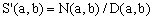, where N(a,b) and D(a,b) are polynomials in a,b. This requirement needs to be complemented by proper dimensional (or scaling) behavior. Since a and b are quantities with the dimension of length, all the terms of each of the two polynomials must have the same total degree and, since S(a,b) has also a dimension of length, the degree of N(a,b) must be higher by 1 than that of D(a,b). In addition to all this, we require the approximations to be invariant with respect to the exchange of a and b, regardless of the numerical values of the adjustable coefficients. This is only possible if, for any admissible pair of exponents, terms such as apbq and aqbp have the same coefficient. Consequently, the algebraic approximations can be classified according to the degree of D(a,b) - henceforth called the order of the algebraic approximation - and they must be of the form (1) with (2), where n is order of the approximation and rk, sk are (n+2) coefficients whose values need to be defined. Any approximation formula for S(a,b) of this form can be rewritten in terms of the ratio y = b/a and leads also to an approximation for the complete elliptic integral E(x): (3), where tk = rk/4 and y2 = 1-x. Since the value of expression (3) does not change when all the coefficients are divided by a common factor, it is possible to normalize it so as s0 = 1. In the following we will assume that this has been done. The number of coefficients to be determined is therefore equal to (n+1). Types of algebraic approximation formulae The set of conditions used to define the coefficients determines what kind of algebraic approximations one obtains. Thus, for example, matching the (n+1) derivatives of E(x) at y = 1 from 0-th to n-th optimizes the formulae for ellipses with low eccentricities and leads to a sub-selection of Padé approximations [1, section K). A similar approach is not possible for flat ellipses with large eccentricities since S(a,b)/4a does not have a convergent Taylor expansion at y = 0 (the behavior . Another family of approximations arises applying the requirement that the formulae be exact for both y = 0 (flat ellipses, perimeter equal to 4a) and y = 1 (round ellipses, perimeter equal to 2πa). The first condition implies t0 = 1, while the second one amounts to (4), a relation which can be used to determine, for example, the highest of the coefficients tk. It is interesting that these same conditions guarantee also a match for the first derivative of S(a,b)/4a at y = 1, while this is not true at y = 0. This explains why perimeters of roundish ellipses with low eccentricities get in any case approximated better than perimeters of flat elipses. With these two constraints, the number of undefined coefficients becomes (n-1) meaning, for example, that there is nothing left to adjust in the case n = 1. When n > 1, one can proceed in two ways. The first one consists in matching the 2nd to (n+1)th derivatives of S(a,b)/4a at y = 1 (0th and 1st derivatives are already matched). In such a case one would obtain Padé-like approximations constrained to the exact value at y = 0. This is reminiscent of the linear Padé combinations introduced in  in order to achieve essentially the same goal (the extent to which the two approaches might be equivalent is at this moment not clear). In the second, numerically more brutal approach, all the (n-1) undefined coefficients are adjusted so as to minimize the maximum relative error of the approximation over the whole interval [0,1] of y values. This prescription defines a unique set of coefficient values and leads to what we will call globally best algebraic approximations or GBA's. One might also consider hybrid algebraic approximations in which one matches m derivatives of S(a,b)/4a at y = 1 and adjusts the remaining (n+1-m) coefficients to minimize the maximum relative error. This leads to a large set of approximations classified according to their order n and their derivative index m = 0, 1, 2, ..., (n+1). In this work, the coefficients of the GBA's (m = 0) were determined for orders n = 1 to 7. The respective error curves are shown in Figure 1 (for graph conventions, consult ).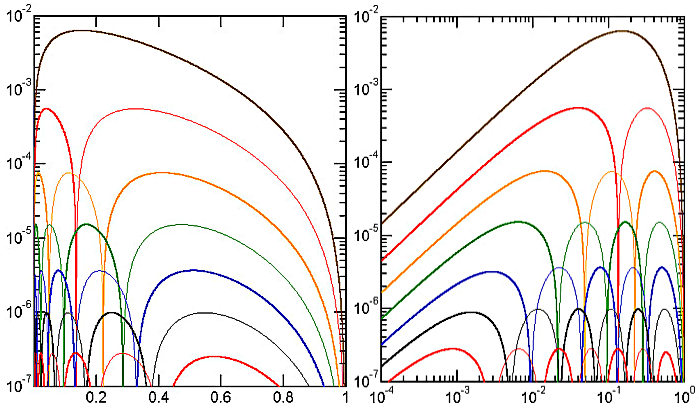Error curves for the globally best algebraic approximations of orders 1 to 7  The horizontal axis corresponds to y = b/a and is either linear (left graph) or logarithmic (right graph). The vertical axis corresponds to the relative error. The error curves of the GBA approximations of various order n are distinguished by color. Their global maximum is highest for n = 1 and smallest for n = 7. The line thickness of each trace is used to indicate the sign of the error: bold sections are positive, thin sections negative. The graphs were produced using the enclosed MATLAB program EllipseAlgApprox.m.   Particular cases Order 1, rel.error < 6313 ppm n =1 is the only case in which there are no freely adjustable coefficients. It coincides with the Cantrell's Particularly Fruitful approximation (see  for a discussion of its origin) and can be written as (A1)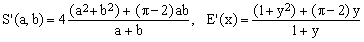. Order 2, rel.error < 557.3 ppm This approximation appears to be new: (A2)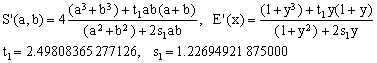. Order 3, rel.error < 75.96 ppm This approximation turns out to be equivalent to Sykora 2005 [1:C5] provided that its coefficients are numerically adjusted as suggested by Cantrell . Using the formalism of this Note, it can be written as (A3)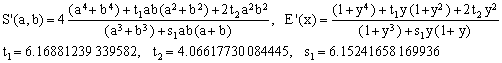. Order 4, rel.error < 15.29 ppm This approximation appears to be new: (A4)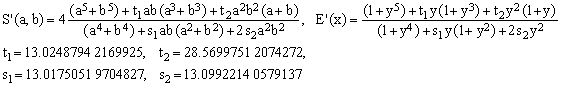. Order 5, rel.error < 3.65 ppm This approximation is equivalent to Cantrell 2006 [2,3] provided that its coefficients are better adjusted. In his original announcement , David Cantrell in fact mentions that he had problems with the numerical minimization and that his coefficients and his relative error bound of 5.2 ppm need not be the best. Using the formalism of this Note, the optimized formula can be written as (A5)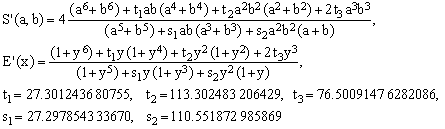. Expanding both this and Cantrell's 2006 formulas as plain rational functions and comparing the coefficients, one obtains the relations between our tk's and sk's and the Cantrell's coefficients s, p, q, r, t. It follows that the optimal values of the latter should have been s = 22.29785433367, t = 33.65830998486, p = 3.98644261166, q = 82.24320600580, r = 84.00260009659. Order 6, rel.error < 0.988 ppm This approximation is new and it is the first one with guaranteed maximum error smaller than 1 ppm: (A6)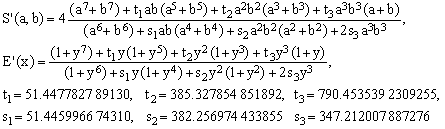. Order 7, rel.error < 0.282 ppm This, of course, is also a new approximation: (A7)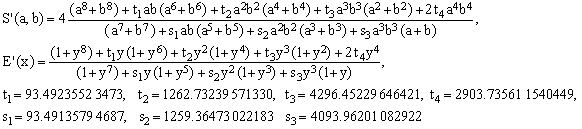. Discussion This Note intentionally avoids the problem of optimizing the approximations from the algorithmic point of view. As presented here, the expressions maintain the elegance originating from a single guiding principle. Minimizing the number of arithmetic operations in order to optimize computational efficiency is another matter which might well hide the fact that the expressions form a single, internally coherent family. The numerical values of the coefficients reported here are those obtained from the optimization process. I have tried hard to make sure that the reported maximum errors be correct and I do not believe one can improve them except, perhaps, beyond the fourth significant decimal digit. The coefficient values, however, do have a certain tolerance range and could be rounded to a lower number of significant digits (maybe two more than the maximum relative error). It is yet to be seen, for example, whether there is a set of single-precision coefficients which would maintain the precision of the 6th and 7th order algebraic approximations. I think that it is probable but I have not investigated the matter in detail. For approximations of 5th and higher orders, the numeric optimization process is very slow and rather unreliable in the sense the local minima due to limited precision and/or discrete error-curve sampling all but preclude a straightforward minimization. I had to resort to alternate minimization of the absolute value of maximum relative error and the mean square of the relative deviations. Such an alternation, reminiscent of simulated annealing, helps to nudge the process out of false local minima and drive it towards the absolute one. It is also helpful to use Eq.(4) to compute the highest tk coefficient rather than t1. Determining the coefficients for still higher-order GBA approximations may be an arduous task (a nice exercise in numeric optimization, though) which I, at least for the moment, refrain from undertaking. Extrapolating the current results, there is no doubt that the 8-th order approximation has a maximum relative error smaller than 0.1 ppm (100 ppb).
 References and links Sykora S., Approximations of Ellipse Perimeters, in Stan's Library, Ed.S.Sykora, Vol I, December 2005. David W. Cantrell, Arithmetic Approximations of the Perimeter of an Ellipse. Sykora S., Approximations of Ellipse Perimeters: Addendum, in Stan's Library, Ed.S.Sykora, Vol I, February 2006. Perimeter of an Ellipse. A Review by Gérard P.Michon. Page updated at a more recent date than this article. Sykora S., Algebraic Approximations of Ellipse Perimeters, in Stan's Library, Ed.S.Sykora, Vol I, June 2006. (URL of this Note).  For more references and links, click here.
 TOP | Other math articles in Stan's Library | SCIENCE Links Stan's Courses | Stan's HUB | TOP